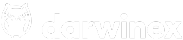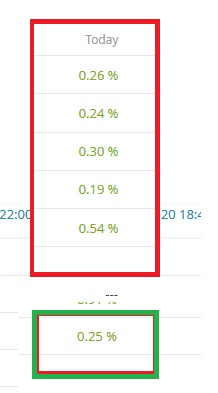CFDs are complex instruments and come with a high risk of losing money rapidly due to leverage. 64 % of retail investor accounts lose money when trading CFDs with this provider. You should consider whether you understand how CFDs work and whether you can afford to take the high risk of losing your money.# Clarifications on portfolio return

Hi

still not understand how the profit is calculated?when I sum my all darwins profit I get 1,53% in return, but in monthly profit I see only 0.25% Why so low?

Try it with average and calculate in currency, exactly:
Sum of (Investment amount for each Darwin multiplied by performance) divided by total investment amount.
Multiply by 100 to get the % value.
Works?

3 Likes

yes, thank you. The perfomance is from all investment, not a sum of all performance

2 Likes